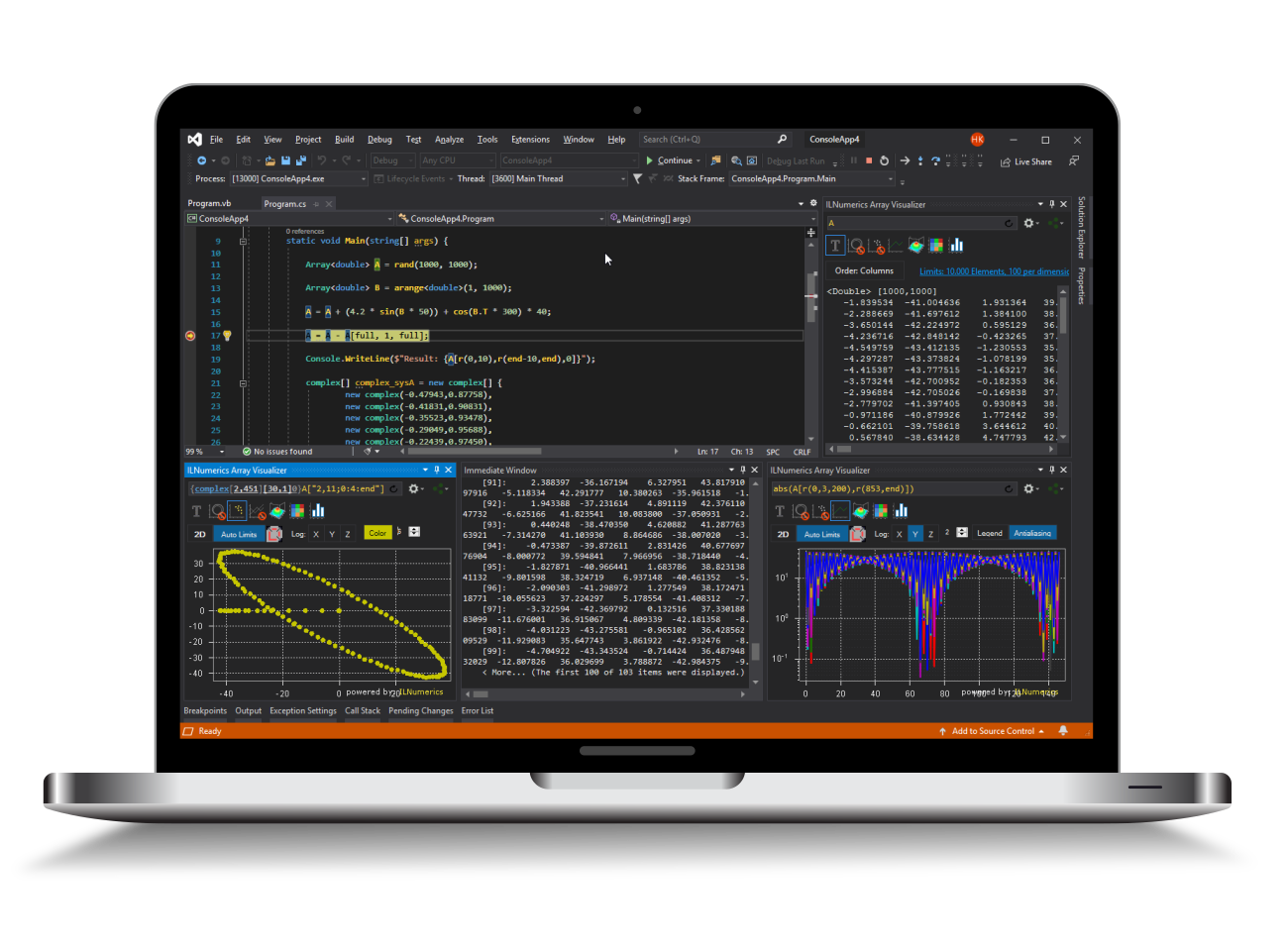Industrial Data Science
in C# and .NET:
Simple. Fast. Reliable.ILNumerics - Technical Computing

Modern High Performance Tools for Technical

Computing and Visualization in Industry and Science

tgt

# The least squares Levenberg-Marquardt method in .NET (C# and Visual Basic)

The Levenberg-Marquardt method is used to do a curve fitting to measured data. It minimizes the expression $$S(\beta) = \sum_i^n ( y_i - f (x_i, \beta) )^2$$ The measured data is collected in the $y_i$ and is measured at the points $x_i$. The function $f$ depends on the parameters $\beta$, which are varied to minimize $S(\beta)$, the error. For more details check out the Wikipedia article.

## A simple example for the Levenberg-Marquardt method

Here is a simple example for a least square problem.

The leastsq_levm routine is a convienient and simple method for least square fits in .NET (C# and Visual Basic). You can find more details about nonlinear least squares and some examples on our website. The class reference for leastsq_lvm can be found here.